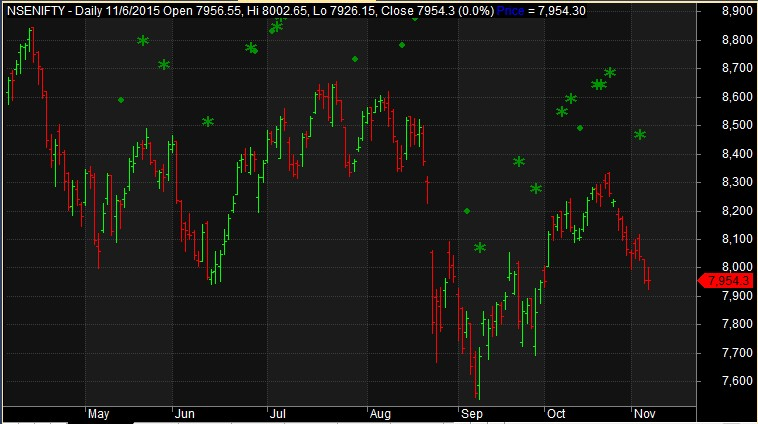# Amibroker AFL: Step by Step Tutorial- Part 3

This is Part 3 of Amibroker AFL tutorial series. If you haven’t already gone through Part 1 & 2 of this series, please refer the below links:-

Amibroker AFL: Step by Step Tutorial- Part 1

Amibroker AFL: Step by Step Tutorial- Part 2

### Plotting a simple EMA Crossover

```//------------------------------------------------------
//  Formula Name:    Simple EMA Crossover
//------------------------------------------------------

_SECTION_BEGIN("Simple EMA Crossover");

SetChartOptions(0,chartShowArrows | chartShowDates);
_N(Title = StrFormat("{{NAME}} – {{INTERVAL}} {{DATE}} Open %g, Hi %g, Lo %g, Close %g (%.1f%%) {{VALUES}}", O, H, L, C, SelectedValue( ROC( C, 1 ) ) ));

FastEMA=Param("FastEMA",20,1,200,1);
SlowEMA=Param("SlowEMA",50,1,200,1);

Buy = Cross(ema( C, FastEMA ),ema( C, SlowEMA ));
Sell =Cross( ema( C, SlowEMA ), ema( C, FastEMA )) ;

Sell = ExRem( Sell, Buy );
Short = Sell;

Plot( Close, "Price", colorBlue, styleCandle );
Plot(ema( C, FastEMA ),"FastEMA",colorWhite);
Plot(ema( C, SlowEMA ),"SlowEMA",colorBlue);

_SECTION_END();

``````//------------------------------------------------------
//
//  Formula Name:    Simple EMA Crossover with Buy Sell Arrows
//------------------------------------------------------

_SECTION_BEGIN("Simple EMA Crossover with Buy Sell Arrows");

SetChartOptions(0,chartShowArrows | chartShowDates);
_N(Title = StrFormat("{{NAME}} – {{INTERVAL}} {{DATE}} Open %g, Hi %g, Lo %g, Close %g (%.1f%%) {{VALUES}}", O, H, L, C, SelectedValue( ROC( C, 1 ) ) ));

FastEMA=Param("FastEMA",20,1,200,1);
SlowEMA=Param("SlowEMA",50,1,200,1);

Buy = Cross(ema( C, FastEMA ),ema( C, SlowEMA ));
Sell =Cross( ema( C, SlowEMA ), ema( C, FastEMA )) ;

Sell = ExRem( Sell, Buy );
Short = Sell;

Plot( Close, "Price", colorBlue, styleCandle );
Plot(ema( C, FastEMA ),"FastEMA",colorWhite);
Plot(ema( C, SlowEMA ),"SlowEMA",colorBlue);

/* Plot Buy and Sell Signal Arrows */
PlotShapes(IIf(Buy, shapeSquare, shapeNone),colorGreen, 0, L, Offset=-40);
PlotShapes(IIf(Cover, shapeSquare, shapeNone),colorGreen, 0, L, Offset=-40);
PlotShapes(IIf(Cover, shapeSquare, shapeNone),colorLime, 0,L, Offset=-50);
PlotShapes(IIf(Cover, shapeUpArrow, shapeNone),colorWhite, 0,L, Offset=-45);
PlotShapes(IIf(Sell, shapeSquare, shapeNone),colorRed, 0, H, Offset=40);
PlotShapes(IIf(Sell, shapeSquare, shapeNone),colorOrange, 0,H, Offset=50);
PlotShapes(IIf(Sell, shapeDownArrow, shapeNone),colorWhite, 0,H, Offset=-45);
PlotShapes(IIf(Short, shapeSquare, shapeNone),colorRed, 0, H, Offset=40);
PlotShapes(IIf(Short, shapeSquare, shapeNone),colorOrange, 0,H, Offset=50);
PlotShapes(IIf(Short, shapeDownArrow, shapeNone),colorWhite, 0,H, Offset=-45);

_SECTION_END();

```### Plotting Inside Day and Outside Day patterns

Note: Circle represents Inside Day and Star represents Outside Day

```//------------------------------------------------------
//  Formula Name:    Inside Day Outside Day Patterns
//------------------------------------------------------

_SECTION_BEGIN("Inside Day Outside Day Patterns");

SetChartOptions(0,chartShowArrows | chartShowDates);
_N(Title = StrFormat("{{NAME}} – {{INTERVAL}} {{DATE}} Open %g, Hi %g, Lo %g, Close %g (%.1f%%) {{VALUES}}", O, H, L, C, SelectedValue( ROC( C, 1 ) ) ));

Plot( Close, "Price", colorBlue, styleBar);
PlotShapes(IIf(Inside(), shapeSmallCircle, shapeNone),colorGreen, 0, H, Offset=100);
PlotShapes(IIf(Outside(), shapeStar, shapeNone),colorGreen, 0, H, Offset=100);

_SECTION_END();

```### Plotting MACD indicator with Signal Line

```//------------------------------------------------------
//  Formula Name:    MACD Crossover
//------------------------------------------------------

_SECTION_BEGIN("MACD Crossover");

SetChartOptions(0,chartShowArrows | chartShowDates);
_N(Title = StrFormat("{{NAME}} - {{INTERVAL}} {{DATE}} Open %g, Hi %g, Lo %g, Close %g (%.1f%%) {{VALUES}}", O, H, L, C ));

Plot( Close, "Price", colorBlue, styleCandle);
fast = Param( "Fast avg", 12, 2, 200, 1 );
slow = Param( "Slow avg", 26, 2, 200, 1 );
signal1 = Param( "Signal avg", 9, 2, 200, 1 );

Plot(MACD(fast,slow),"MACD",color=colorRed,styleOwnScale);
Plot(Signal(fast,slow,signal1),"Signal",color=colorBlue,styleOwnScale);

Sell= Cross(Signal(fast,slow,signal1),MACD(fast,slow));
Sell = ExRem( Sell, Buy );
Short = Sell;

_SECTION_END();

```### Plot Price level at Buy/Sell signal

```//------------------------------------------------------
//
//  Formula Name:    Plot Price Level
//  E-mail:
//  Website:
//------------------------------------------------------

_SECTION_BEGIN("Plot Price Level");

SetChartOptions(0,chartShowArrows | chartShowDates);
_N(Title = StrFormat("{{NAME}} – {{INTERVAL}} {{DATE}} Open %g, Hi %g, Lo %g, Close %g (%.1f%%) {{VALUES}}", O, H, L, C, SelectedValue( ROC( C, 1 ) ) ));

FastEMA=Param("FastEMA",20,1,200,1);
SlowEMA=Param("SlowEMA",50,1,200,1);

Buy = Cross(ema( C, FastEMA ),ema( C, SlowEMA ));
Sell =Cross( ema( C, SlowEMA ), ema( C, FastEMA )) ;

Sell = ExRem( Sell, Buy );
Short = Sell;

Plot( Close, "Price", colorBlue, styleCandle );
Plot(ema( C, FastEMA ),"FastEMA",colorWhite);
Plot(ema( C, SlowEMA ),"SlowEMA",colorBlue);
dist = 1.5*ATR(10);
for( i = 0; i < BarCount; i++ )
{
if( Buy[i] ) PlotText( "Buy\n@" + C[ i ], i, L[ i ]-dist[i], colorGreen,colorYellow );
if( Sell[i] ) PlotText( "Sell\n@" + C[ i ], i, H[ i ]+dist[i], colorRed, colorYellow );
}

_SECTION_END();

```#### Related Posts

1.Gautam

Dear Sir,
I am very pleased by your efforts to put these things in simplest way. Its very easy to understand for nor coding peoples like me.
Bye the way, when I copy the formula for “Plot Price level at Buy/Sell signal” afl, I got error message in line 28/28, 28/34 & 31/11. Please do needful.
Thanks again.

2.admin

Hello Gautam,
Thanks for your kind words. We would definitely look into the error you pointed out. Meanwhile can you please let me know which version of Amibroker you are using?

•Gautam

Sir, its 5.8

3.Gautam

Sorry, Its 5.7

•admin

Hello Gautam,
We have corrected the code. Please check now. Thanks a lot for bringing this up to our attention.

4.Rishav

Very ardent effort to simplify lives in trading.
Cheers!

•admin

Thanks Rishav!

5.Paul

With Version 6.00.2 says, there are still errors with “Plot Price level at Buy/Sell signal” afl.

•admin

Corrected. Thanks!

6.Paul

Should this line for( i = 0; i < BarCount; i++ ) be for( i = 0; i < BarCount; i++ ) ? If so, how do I execute it to have the graph above in Amibroker platform?

7.Jai

can u kindly guide me on the following:
1. how to plot date and time at buy sell signal,

thanks

8.Sunil Kalore

Great work for new traders , thank you very much.

9.Sunil Kalore

I want more Trading Tutorial , thank you